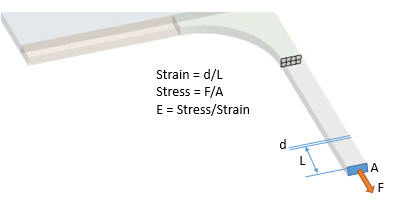## General Mechanical

Topics relate to Mechanical Enterprise, Motion, Additive Print and more

•jinglun
Subscriber

Hi, I am simulating the tensile test of a material with these properties. To take note of is the X Young's modulus of 26.9 GPa.

•peteroznewman
Subscriber
nYoung's Modulus is an input to the model, so you can be sure that you are measuring the wrong things to show the input value by calculation from results in the model.nTensile testing is based on a Gauge Length L, which is a length a long way from the blend that widens the thin section out to the wide section. The change in length of the Gauge Length is measured as d. A load cell measures the force F and the cross-sectional area A of the Gauge section is know. Calculate the Youngs Modulus E using the formula shown below.nI assume you have a Displacement BC at the center where the deformation along x is exactly zero and all other DOF are Free. Slice the solid model one more time to get a known Gauge length a distance L from the plane of symmetry. Then you can get results for the X displacement of a vertex on the new cut plane to get d. I assume you are using the Share button on the Workbench tab of SpaceClaim. Use CAD to measure the area of the cross-section A. In Mechanical, Probe the Reaction Force for the Displacement X=0 on the YZ plane of symmetry to get F.n